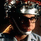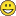# QlikView App Dev

Discussion Board for collaboration related to QlikView App Development.

Announcements
Live chat with experts, bring your API Integration questions. June 15th, 10 AM ET. REGISTER TODAY
cancel
Showing results for
Search instead for
Did you mean:Not applicable

## "If" with a variable

Hi,

I hope you will understand my problem, I don't speak english very well !

I work on Qlikview 10, and I don't understand how works variables in a "if". I have a input box with a list of indicator name stored in a variable as text ([Nombre de bénéficiaires];nombre d’actes;[Montant de la dépense]...) so that the user can change it to see what he wants.

In one of the graphics, I need to do something different if the indicator name is "[Nombre de bénéficiaires]", I tried this but it doesn't work :

`if(\$(vSelectionGraph2)='[Nombre de bénéficiaires]', if(GetSelectedCount([Région du PS]) = 0 and GetSelectedCount([Département du PS]) = 0 and GetSelectedCount([Spécialité du PS]) > 0,sum({\$<[Type de fichier]={'2_F14'}>}NB_BEN),if(GetSelectedCount([Région du PS]) >0 or GetSelectedCount([Département du PS]) >0 and GetSelectedCount([Spécialité du PS]) > 0,sum({\$<[Type de fichier]={'2_F03'}>}NB_BEN))),if(\$(vSelectionGraph2)<>'Nombre de bénéficiaires',sum({\$<[Type de fichier]={'3_F01'}>}\$(vSelectionGraph2))))`

It only works with the last part if (\$(vSelectionGraph2)<>'Nombre de bénéficiaires'....), so I think that "\$(vSelectionGraph2)='[Nombre de bénéficiaires]'" is the problem. But I don't understand why, I thought that \$(variable) was replaced by the value, and in fact it seems to do that in the sum function at the end of my code.

Can someone help me ?

Thank you.

1 Solution

Accepted SolutionsMVP

Hi

I understand your English just fine, but I am not sure I understand the expression you posted. You start with

if(\$(vSelectionGraph2)='Nombre de bénéficiaires',

which implies that vSelectionGraph2 is a string, but you end with

Sum({...} \$(vSelectionGraph2))))

which requires vSelectionGraph2 to be number. Is vSelectionGraph2 a field name? If that is so, then

`if('\$(vSelectionGraph2)'='Nombre de bénéficiaires',           if(GetSelectedCount([Région du PS]) = 0 and GetSelectedCount([Département du PS]) = 0 and GetSelectedCount([Spécialité du PS]) > 0,          sum({\$<[Type de fichier]={'2_F14'}>} NB_BEN),                    if(GetSelectedCount([Région du PS]) >0 or GetSelectedCount([Département du PS]) >0 and GetSelectedCount([Spécialité du PS]) > 0,                    sum({\$<[Type de fichier]={'2_F03'}>} NB_BEN))), if('\$(vSelectionGraph2)'<>'Nombre de bénéficiaires',          sum({\$<[Type de fichier]={'3_F01'}>} \$(=\$(vSelectionGraph2)))))`

.. might do the trick. That corrects the compare problem (note the quotes around \$(vSelectionGraph2)) and will evaluate \$(vSelectionGraph2) as a field name.

Actually, I think your second comparison is redundant. This is the same:

`if('\$(vSelectionGraph2)'='Nombre de bénéficiaires',           if(GetSelectedCount([Région du PS]) = 0 and GetSelectedCount([Département du PS]) = 0 and GetSelectedCount([Spécialité du PS]) > 0,          sum({\$<[Type de fichier]={'2_F14'}>} NB_BEN),                    if(GetSelectedCount([Région du PS]) >0 or GetSelectedCount([Département du PS]) >0 and GetSelectedCount([Spécialité du PS]) > 0,                    sum({\$<[Type de fichier]={'2_F03'}>} NB_BEN))), sum({\$<[Type de fichier]={'3_F01'}>} \$(=\$(vSelectionGraph2))))`

Hope that helps

Jonathan

Logic will get you from a to b. Imagination will take you everywhere. - A Einstein
8 RepliesNot applicable
Author

Try removing the square brackets '[' around the actual text i.e., your expression should look like this:

\$(vSelectionGraph2)='Nombre de bénéficiaires'

Hope it helpsNot applicable
Author

I tried it, this didn't change anything. But thank you for your answer.

I really don't understand why Qlikview replace \$(vSelectionGraph2) by the right value in the sum function but not in   the if part.Creator

Try removing the dollar sign expansion, just use variable name.

vSelectionGraph2='Nombre de bénéficiaires'Creator

Actually I read the question wrong I thought you wanted to see this in a textbox.

I would break your if down into small parts and see which piece actually doesn't work, starting at the beginning or the code you are asking about now, it may be a case where you are falling through the branches, the reason I say that is because of this statement

'It only works with the last part if (\$(vSelectionGraph2)<>'Nombre de bénéficiaires'....),'Not applicable
Author

Puoi postare un esempioNot applicable
Author

Sadly, it's still not working.But thank you.MVP

Hi

I understand your English just fine, but I am not sure I understand the expression you posted. You start with

if(\$(vSelectionGraph2)='Nombre de bénéficiaires',

which implies that vSelectionGraph2 is a string, but you end with

Sum({...} \$(vSelectionGraph2))))

which requires vSelectionGraph2 to be number. Is vSelectionGraph2 a field name? If that is so, then

`if('\$(vSelectionGraph2)'='Nombre de bénéficiaires',           if(GetSelectedCount([Région du PS]) = 0 and GetSelectedCount([Département du PS]) = 0 and GetSelectedCount([Spécialité du PS]) > 0,          sum({\$<[Type de fichier]={'2_F14'}>} NB_BEN),                    if(GetSelectedCount([Région du PS]) >0 or GetSelectedCount([Département du PS]) >0 and GetSelectedCount([Spécialité du PS]) > 0,                    sum({\$<[Type de fichier]={'2_F03'}>} NB_BEN))), if('\$(vSelectionGraph2)'<>'Nombre de bénéficiaires',          sum({\$<[Type de fichier]={'3_F01'}>} \$(=\$(vSelectionGraph2)))))`

.. might do the trick. That corrects the compare problem (note the quotes around \$(vSelectionGraph2)) and will evaluate \$(vSelectionGraph2) as a field name.

Actually, I think your second comparison is redundant. This is the same:

`if('\$(vSelectionGraph2)'='Nombre de bénéficiaires',           if(GetSelectedCount([Région du PS]) = 0 and GetSelectedCount([Département du PS]) = 0 and GetSelectedCount([Spécialité du PS]) > 0,          sum({\$<[Type de fichier]={'2_F14'}>} NB_BEN),                    if(GetSelectedCount([Région du PS]) >0 or GetSelectedCount([Département du PS]) >0 and GetSelectedCount([Spécialité du PS]) > 0,                    sum({\$<[Type de fichier]={'2_F03'}>} NB_BEN))), sum({\$<[Type de fichier]={'3_F01'}>} \$(=\$(vSelectionGraph2))))`

Hope that helps

Jonathan

Logic will get you from a to b. Imagination will take you everywhere. - A EinsteinNot applicable
Author

vSelectionGraph2 is a text variable with the name of several indicators that the user will choose in a list in a input (for example number of patients,amount...). Then if that indicator is '`Nombre de bénéficiaires`' I have to pick the right value in a particular type of file.

Else, I go in another type of file and the name of the indicator is the same as the value of vSelectionGraph2 . (if the user choose "amount" in the list, vSelectionGraph2 = "amount", and the field with the value I need in my Qlikview QVD file is also "amount").

And what your answered seems to be working, thank you so much !